# Angle Bisector

"The kite rises highest against the wind, not with it."

Isn't this an inspiring thought?

Yes, indeed! On this page, we will be focusing more on the kite, than how it flies. To be more precise, we will be learning about angle bisectors, how to construct it, its theorems, and other interesting properties and facts around angle bisectors.

You can check out the playful calculator to know more about the lesson and try your hand at solving a few interesting practice questions at the end of the page.

Let’s start!## What is Angle Bisector?

Let's have a closer look at the kite. Are you able to see angles being formed at each of its corner?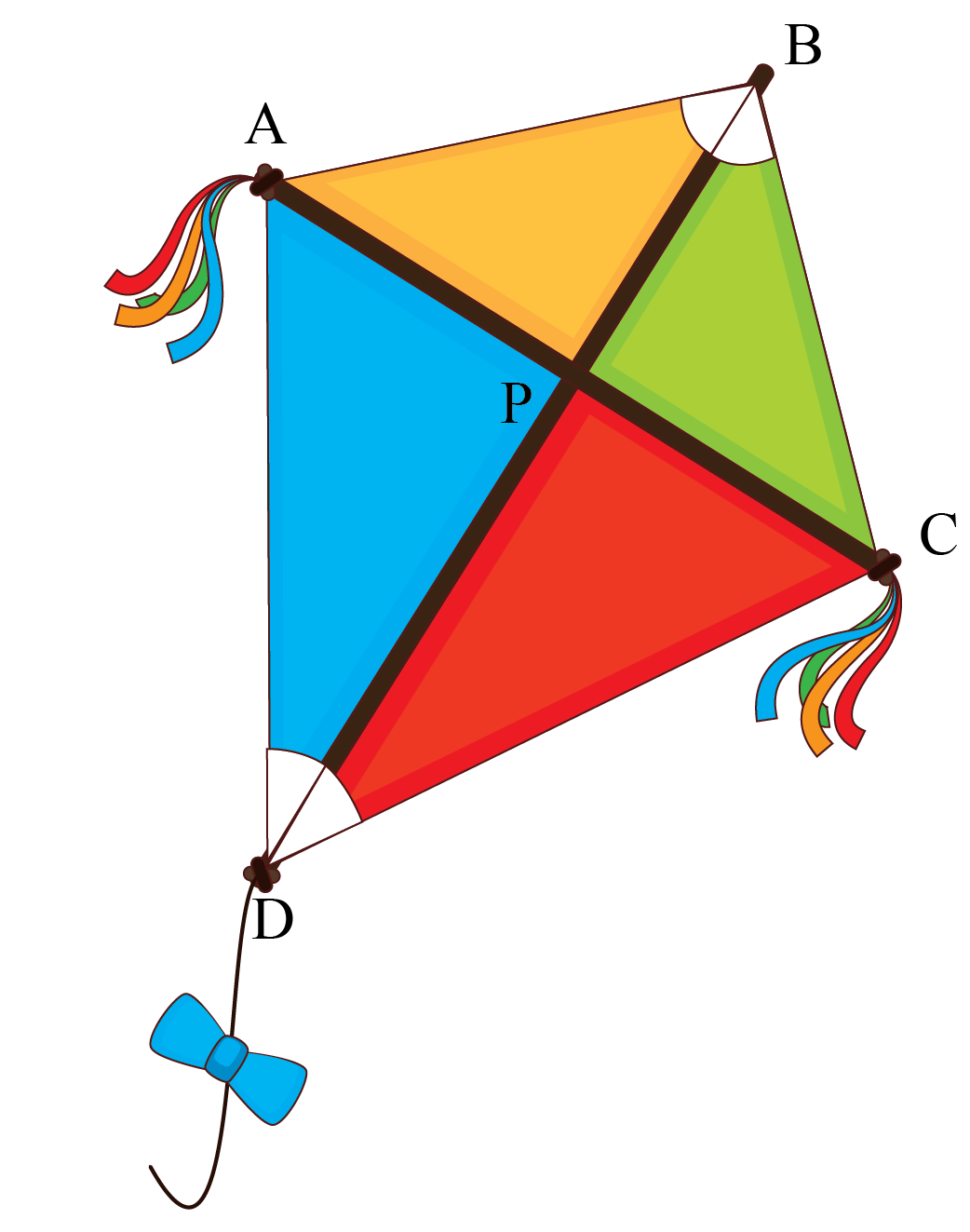In the figure, we can observe that segment BD has divided $$\angle B$$ and  $$\angle D$$ into equal parts.

That's called an angle bisector.

An angle bisector is defined as a ray which divides a given angle into two angles with equal measures.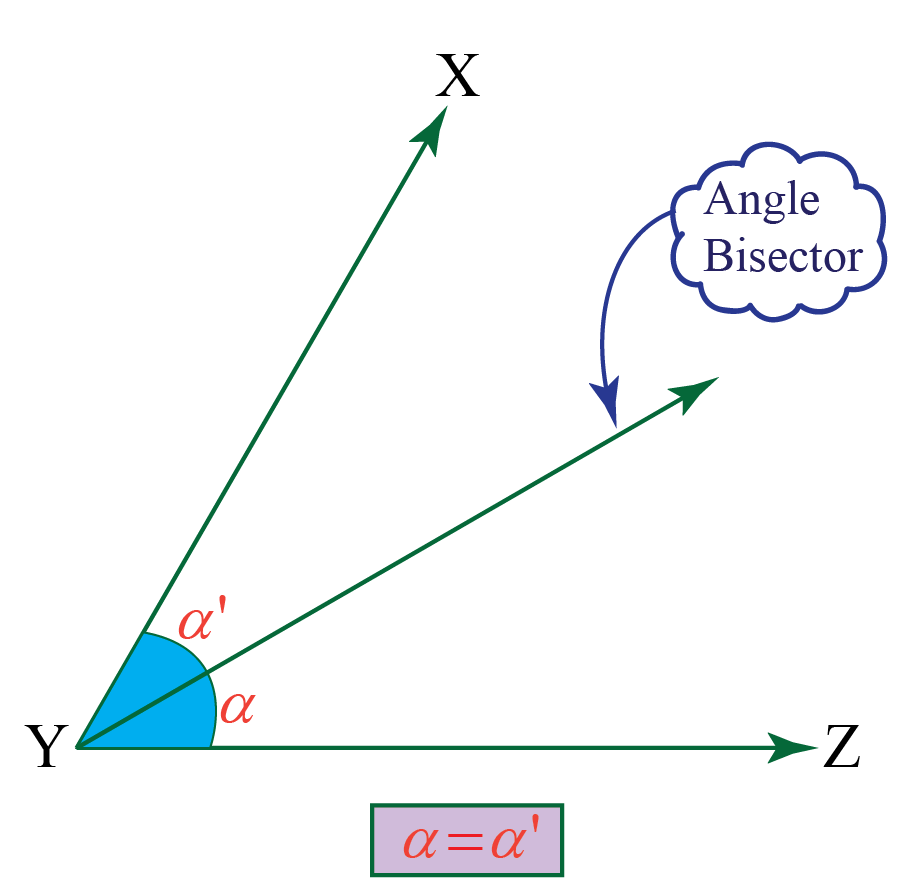Observe below the angle bisector calculator. Try changing measure of $$\angle XYZ$$ and observe the values of $$\angle XYA$$ and $$\angle ZYA$$.

### Properties of an Angle Bisector

1.  Any point on the bisector of an angle is equidistant from the sides of the angle.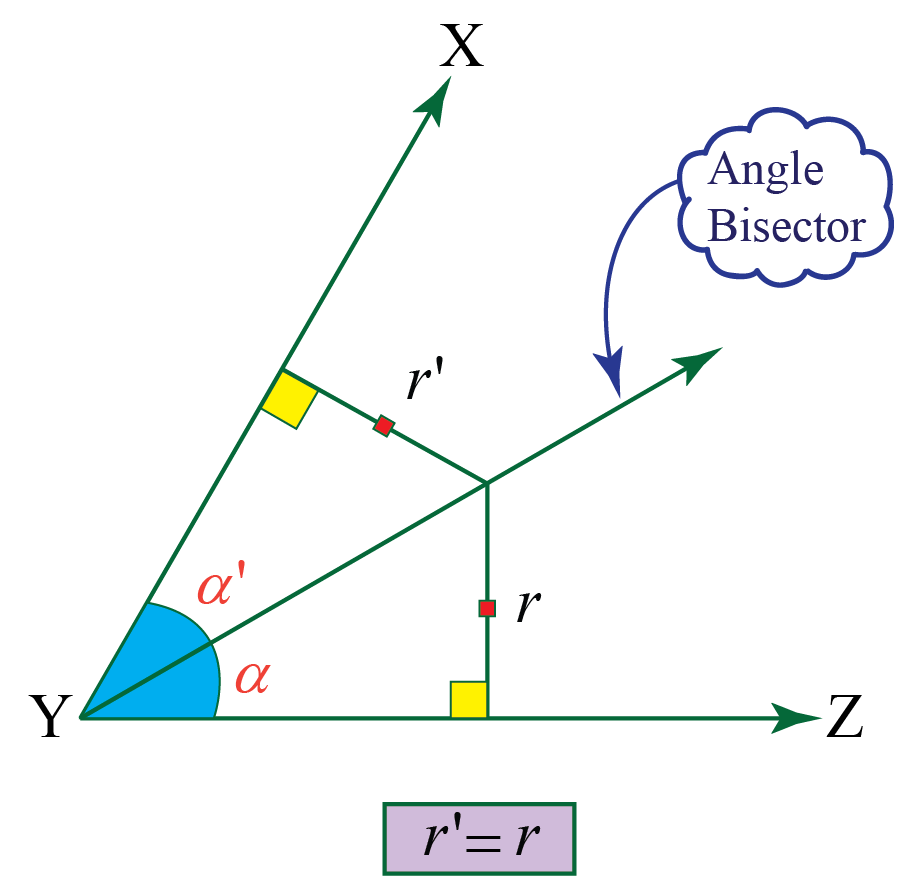2. In a triangle, the angle bisector divides the opposite side in the ratio of the adjacent sides.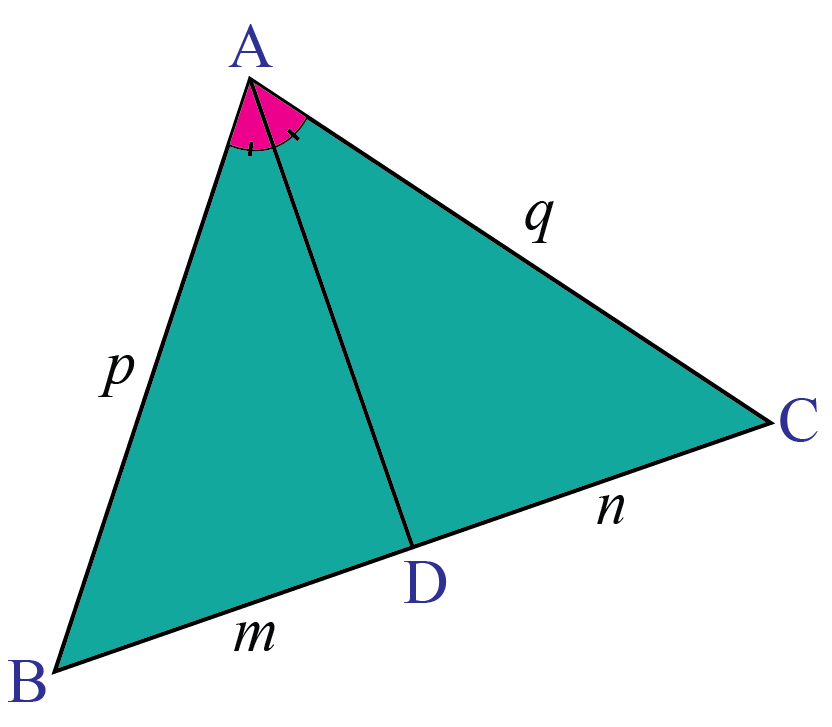This property is called as the angle bisector theorem.

We will study about this property in detail later.

## How to Construct an Angle Bisector?

Let's try constructing the angle bisector for an angle.

In this section, we will see the steps to be followed for angle bisector construction. Let's begin!

### Steps to Construct an Angle Bisector

Step 1: Draw any angle; let's label it as $$\angle ABC.$$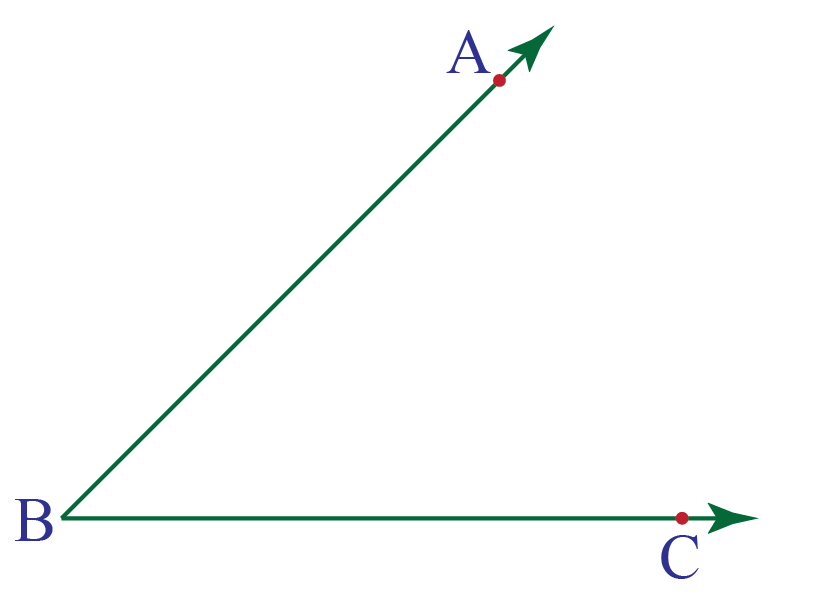Step 2: Taking B as the center and any appropriate radius, draw an arc to intersect the rays BA and BC at, say, E and D respectively. (Refer to the figure below)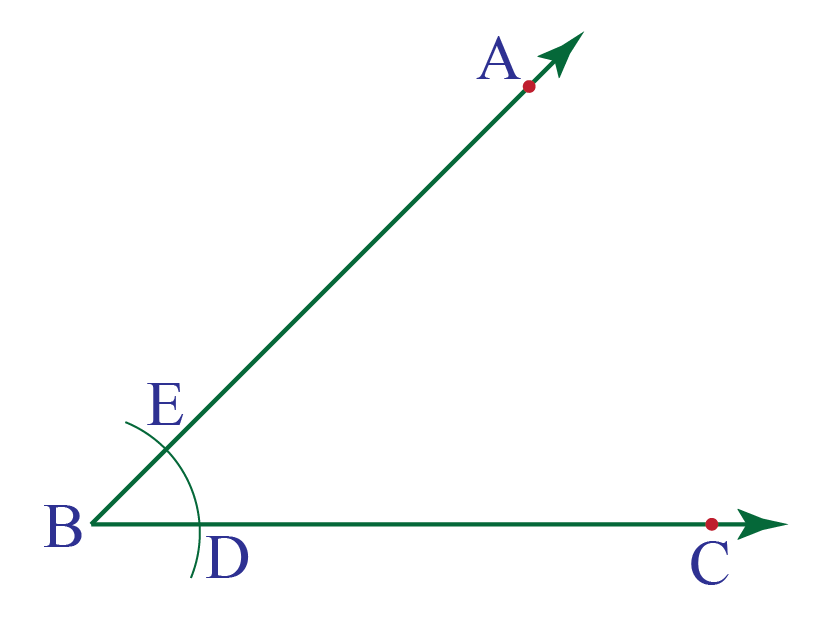Step 3: Now, taking D and E as centers and with radius more than half of DE, draw an arc to intersect each other at F.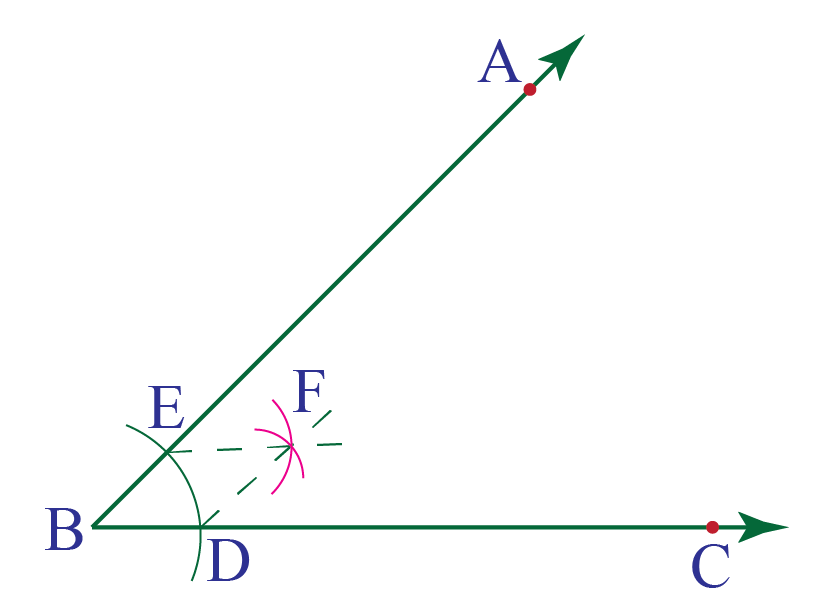Step 4: Draw ray BF. This ray BF is the required angle bisector of angle ABC.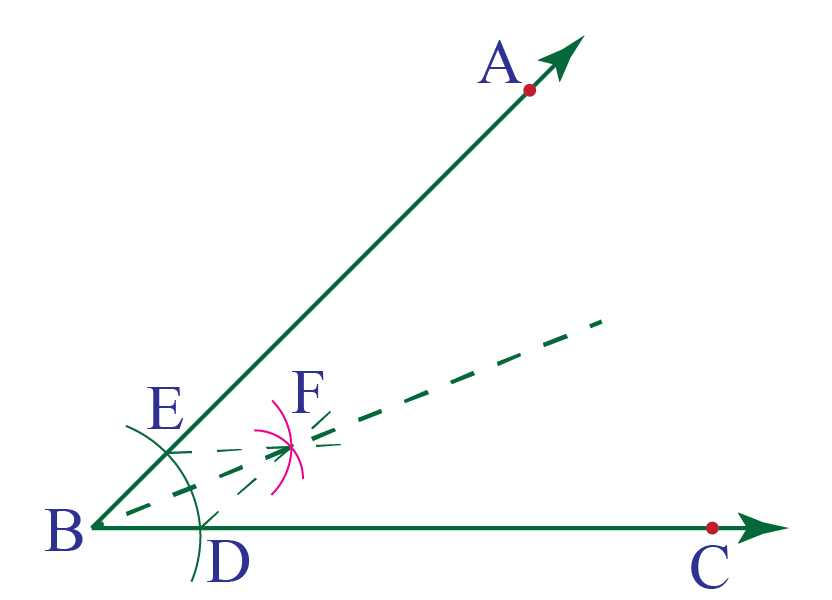## Angle Bisector Theorem

Let's now understand in detail an important property of angle bisector of a triangle as stated in the previous section and then solve angle bisector problems.

This property is known as the Angle Bisector Property of Triangle.

Statement:

An angle bisector of a triangle divides the opposite side into two segments that are proportional to the other two sides of the triangle.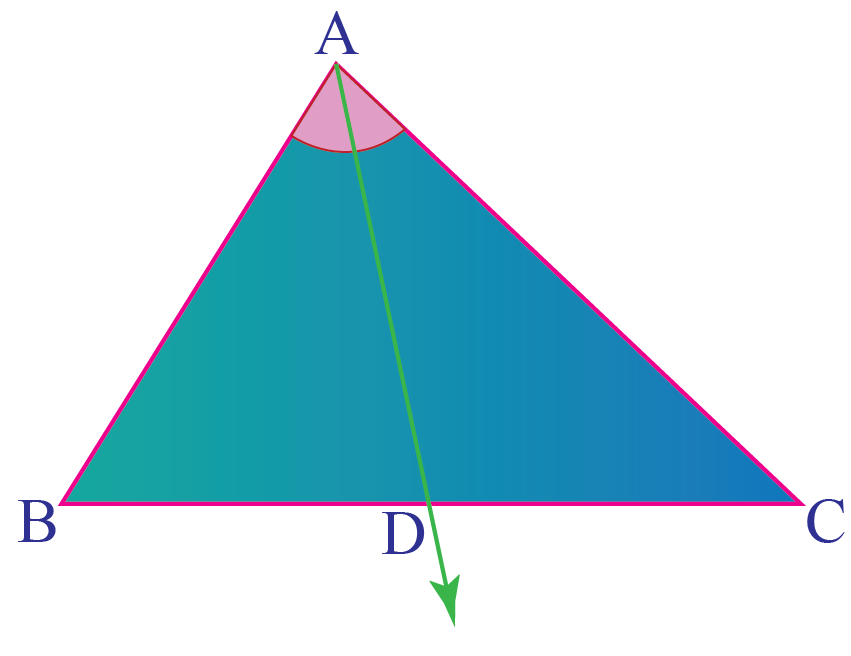Given :

$$\Delta$$ ABC ; AD bisects  $$\angle BAC$$.

To prove

$$\dfrac{AB}{AC}= \dfrac{BD}{DC}$$

Construction

We will prove this result using properties of parallel lines and similarity of triangles.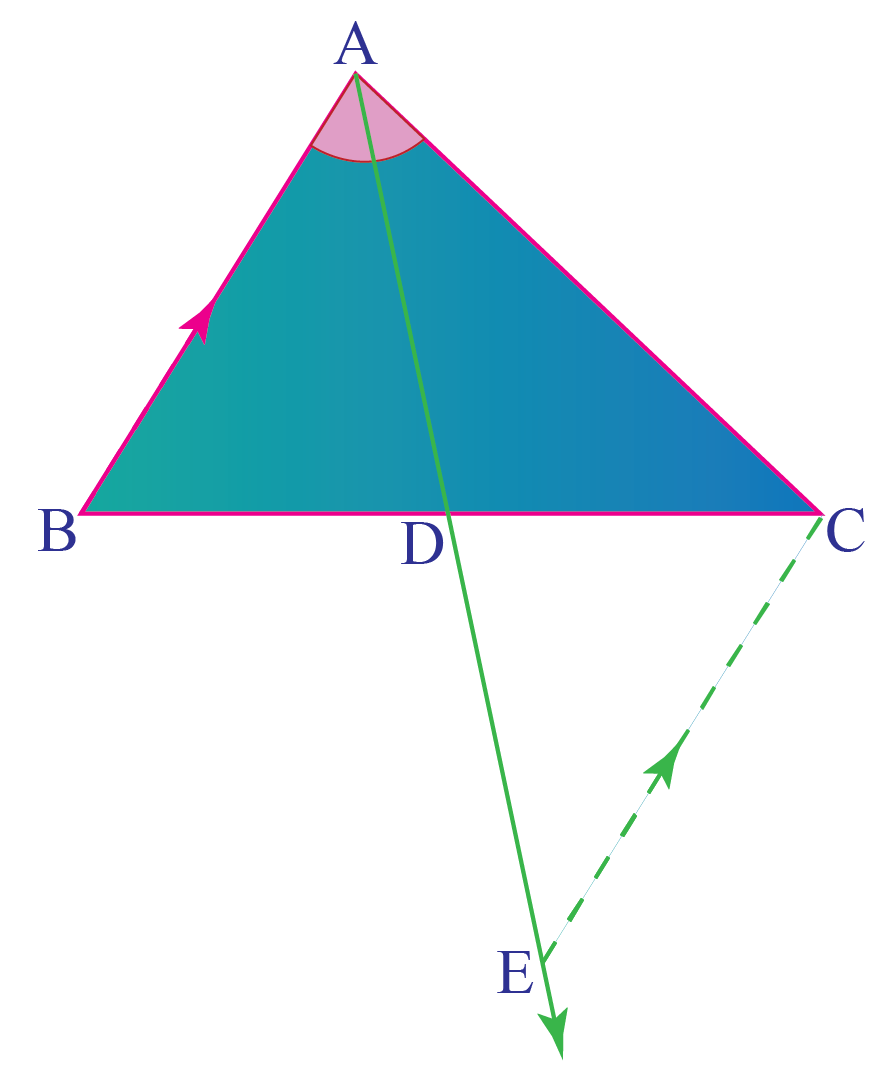We construct an auxiliary line passing through C and parallel to side AB, intersecting AD extended at point E.

### Proof

Step 1: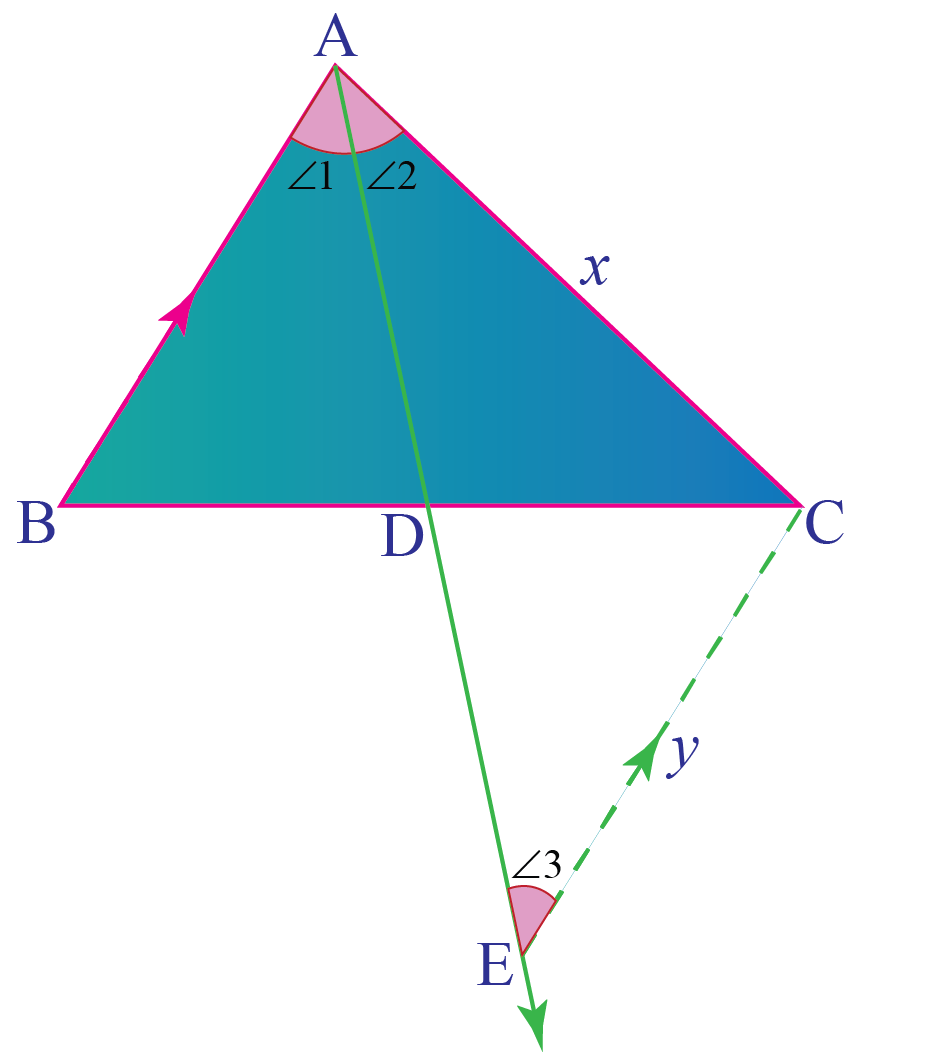As $$AB\left | \right |CE$$,

$$\angle 1=\angle3$$          ( $$\because$$ alternate angles)

Step 2:

But,

$$\angle 1=\angle2$$             ( $$\because$$ AD is angle bisector)

Step 3:

From, Steps (1) and (2) we get,

\begin{align} \angle2 &=\angle3\\\Rightarrow \Delta \text { ACE is }&\text {an isosceles triangle}\\\Rightarrow  x& =y\end{align}

Step 4: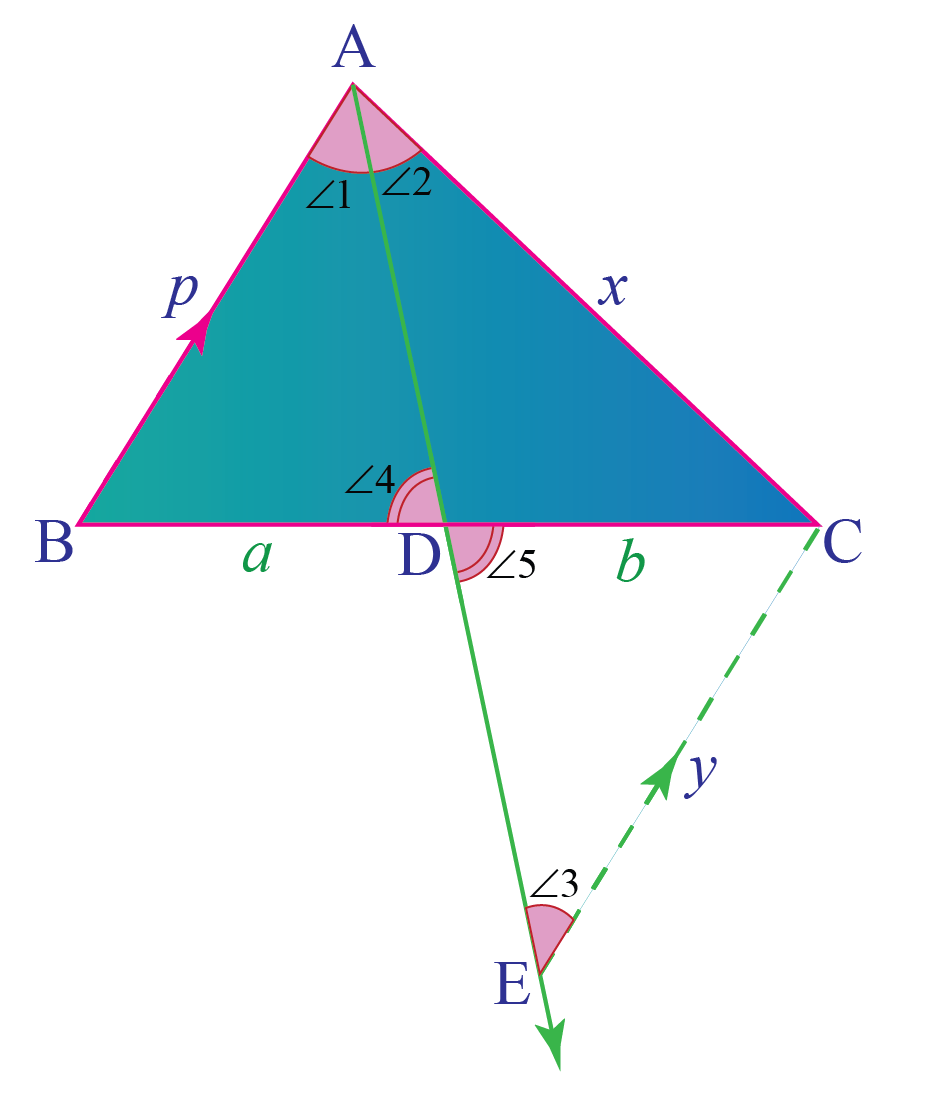Now,

\begin{align} \Delta ABD\sim&\Delta ECD \text {(ByAA test of similarity)}\end{align}
\begin{align}\therefore \dfrac{AB}{EC}&=\dfrac{BD}{CD}\end{align}
\begin{align}\text {(corresponding angle of similar triangles)}\end{align}
\begin{align}\dfrac{p}{y}&=\dfrac{a}{b}\\ \end{align}

Step 5:

So from step 4, we got

$$\hspace{1cm}\dfrac{p}{y}=\dfrac{a}{b}$$

Also from step 3, we have

\begin{align}x &= y\\\Rightarrow  \dfrac{p}{x}&=\dfrac{a}{b}\\i.e. \dfrac{AB}{AC}&=\dfrac{BD}{DC}\end{align}Think Tank
1.The above proof uses similarity of triangle.
Is it possible to prove 'Angle bisector theorem' using Thales theorem (also known as side splitter theorem)?
2. Can you find the length of an angle bisector in a triangle? What is the minimum data required to find the length of an angle bisector?

## Solved Examples

 Example 1

In the figure given below, BD is bisector of $$\angle ABC$$ and BE bisects $$\angle ABD$$.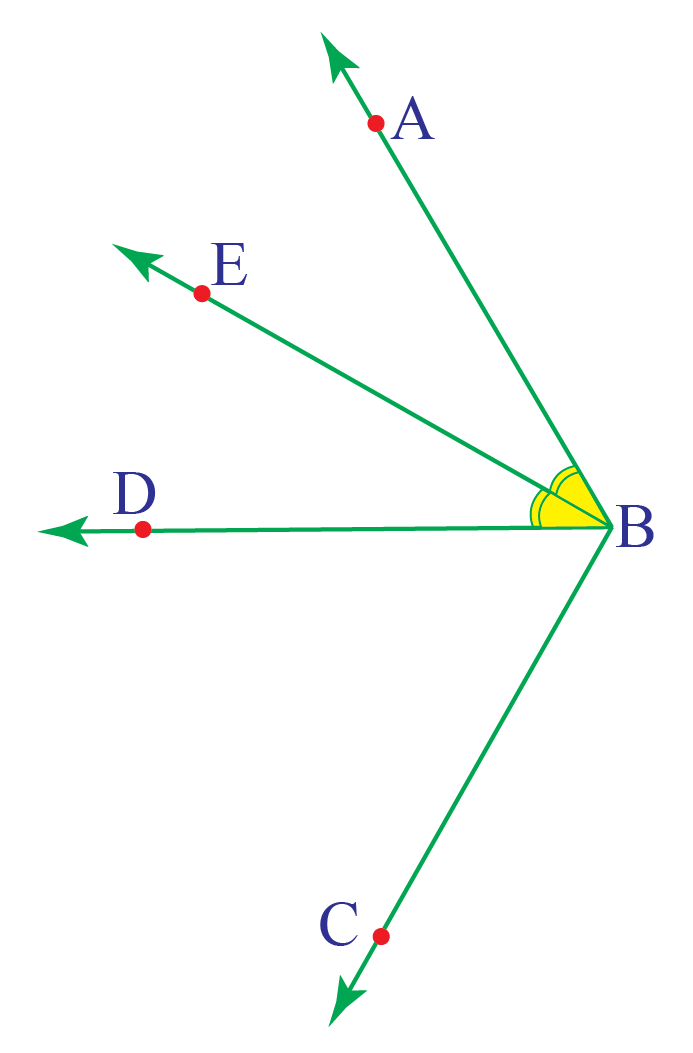Find the measure of $$\angle DBE$$ given that $$\angle ABC= 80^{\circ}$$.

Solution

It is given that $$\angle ABC= 80^{\circ}$$.

\begin{align}\angle ABD &= \dfrac{1}{2} \times \angle ABC\\ &=\dfrac{1}{2} \times 80^{\circ}\\&=40^{\circ}\\Now, \angle DBE&= \dfrac{1}{2} \times \angle ABD\\&=\dfrac{1}{2} \times 40^{\circ}\\&=20^{\circ}\end{align}

 $$\therefore$$ $$\angle DBE= 20^{\circ}$$
 Example 2

In the figure, Ray OD is the angle bisector. Find x.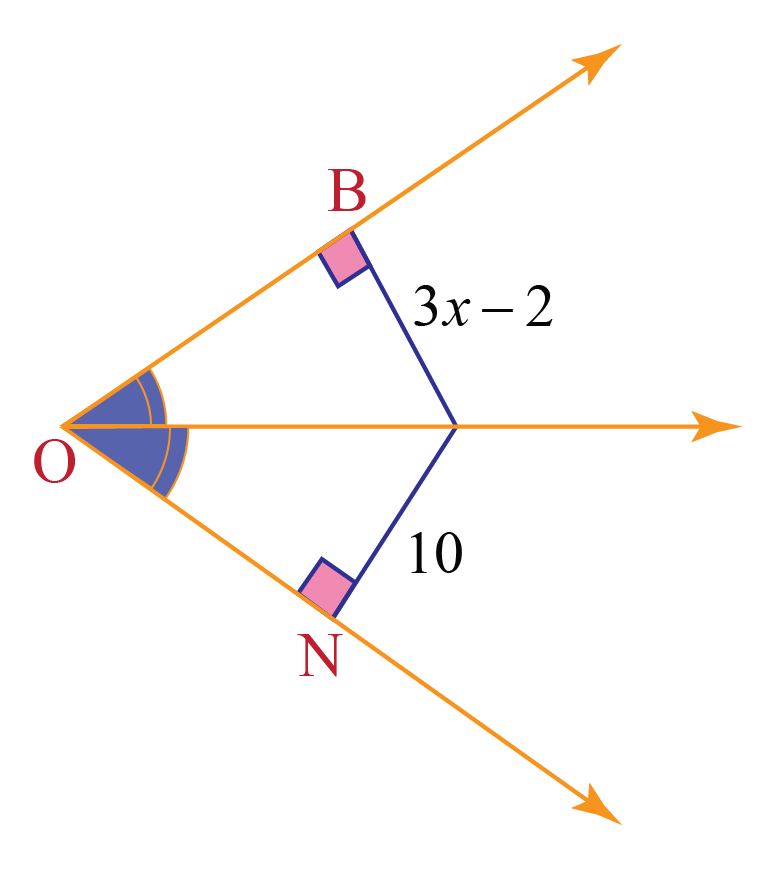Solution

To find x, we will be using the property: Any point on the bisector of an angle is equidistant from the sides of the angle.

So, the point D which lies on the bisector OD will be equidistant from sides OB and ON.

\begin{align} \therefore DB&=DN\\ 3x-2 &=10\\3x&=2+10\\3x&=12\\x&=4\end{align}

 $$\therefore$$ x = 4
 Example 3

If QS is the bisector of $$\angle PQR$$, find x.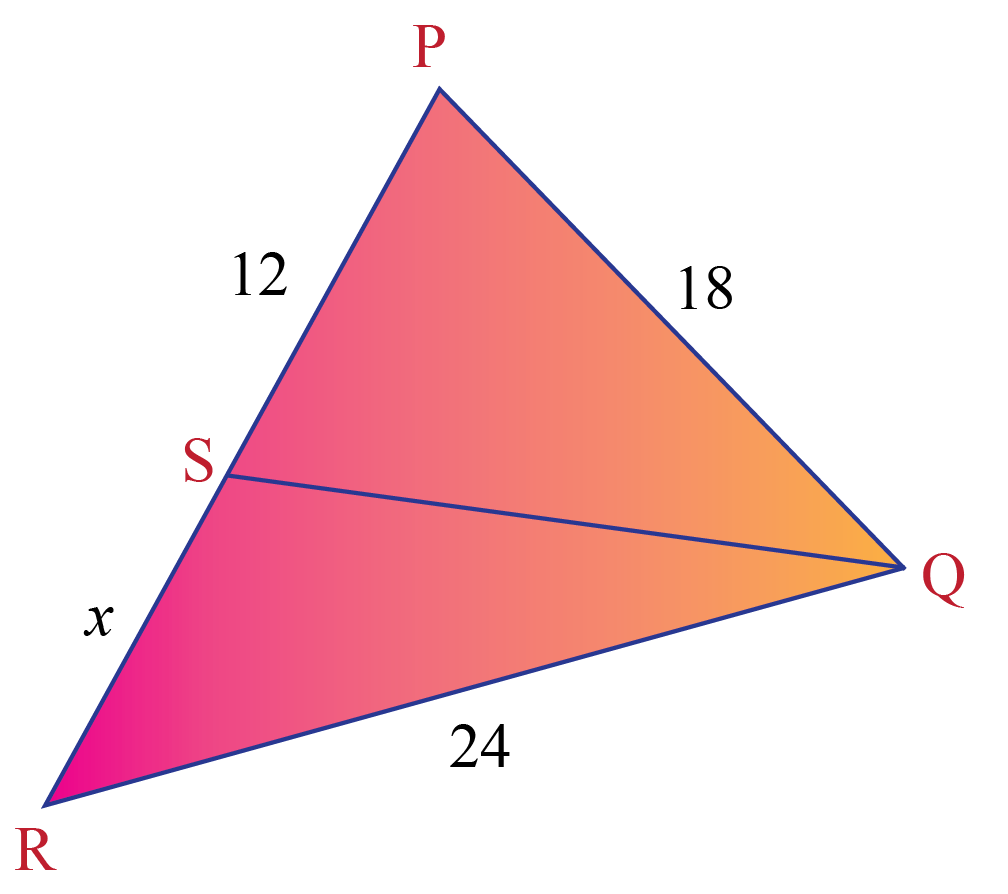Solution

As QS bisects $$\angle PQR$$, by angle bisector theorem we get,

\begin{align} \dfrac{QP}{QR}&=\dfrac{PS}{RS}\\\dfrac{18}{24}&=\dfrac{12}{x}\\\therefore x &=\dfrac{12 \times 24}{18}\\&= \dfrac{2 \times 24}{3}\\&=2\times 8\\&=16\end{align}

 $$\therefore$$ x = 16
 Example 4

From the information given in the diagram below, can we conclude that BD is bisector of $$\angle ABC$$? Justify.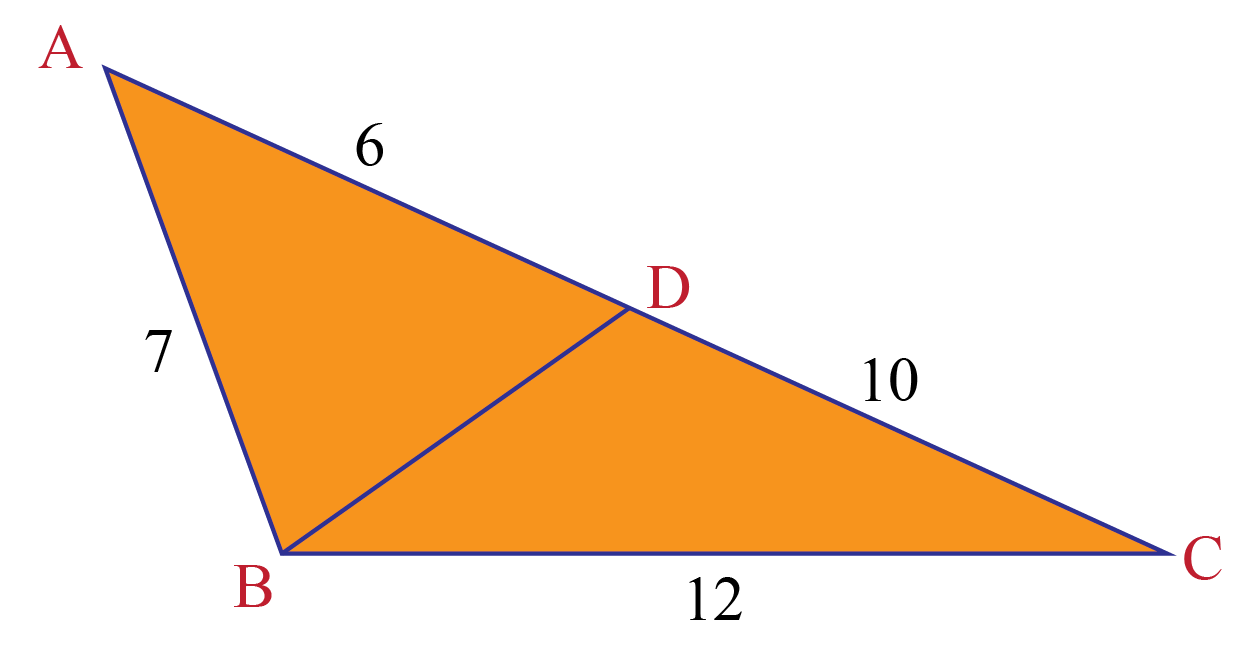Solution

If BD is the angle bisector, then it must satisfy the property

$$\dfrac{BA}{BC}=\dfrac{DA}{DC}$$

Let's calculate these two ratios separately and check if they are equal or not.

\begin{align} L.H.S&= \dfrac{BA}{BC}\hspace{2cm}R.H.S= \dfrac{DA}{DC}\\&= \dfrac{8}{12}\hspace{3.6cm}= \dfrac{6}{10}\\&= \dfrac{2}{3}\hspace{3.8cm}= \dfrac{3}{5}\end{align}

We observe that,

$$L.H.S\neq R.H.S$$

That means BD doesn't satisfy angle bisector theorem.

 $$\therefore$$ BD is not the bisector of $$\angle ABC$$.Challenging Questions

In the figure, PT and QS are angle bisectors. What is the value of y?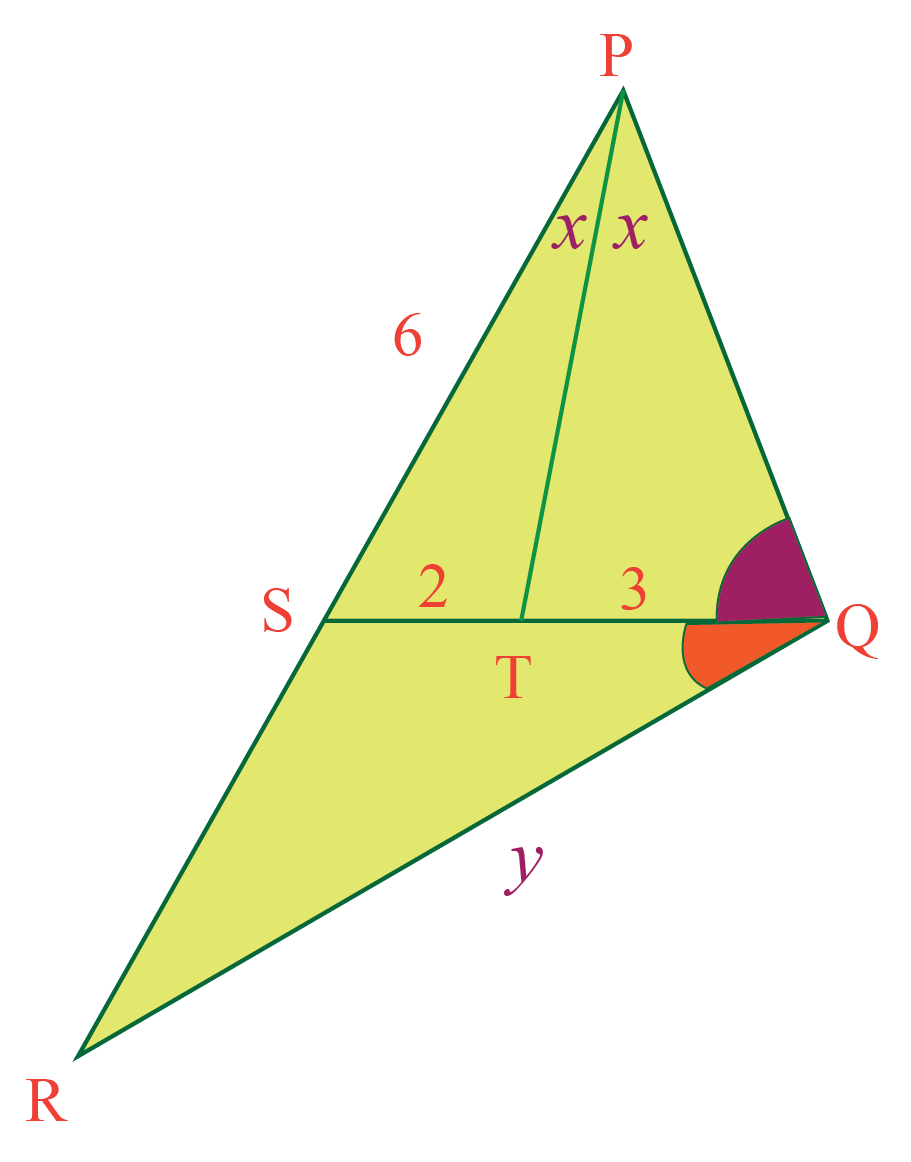## Interactive Questions

Here are a few activities for you to practice.

Select/Type your answer and click the "Check Answer" button to see the result.

## Let's Summarize

We hope you enjoyed learning about the Angle Bisector with the simulations and practice questions. Now you will be able to easily solve problems on angle bisector calculator, angle bisector problems, length of angle bisector, and angle bisector construction.

## About Cuemath

At Cuemath, our team of math experts is dedicated to making learning fun for our favorite readers, the students!

Through an interactive and engaging learning-teaching-learning approach, the teachers explore all angles of a topic.

Be it worksheets, online classes, doubt sessions, or any other form of relation, it’s the logical thinking and smart learning approach that we, at Cuemath, believe in.

## 1. What are properties of angle bisector?

An angle bisector has two properties.

a. Any point on the bisector of an angle is equidistant from the sides of the angle.

b. In a triangle the angle bisector divide the opposite side in the ratio of the adjacent sides.

## 2. Does angle bisector cut an angle in half?

Yes, an angle bisector divides the given angle into two equal angles.

In other words we can say that the measure of these angles will be half of original angle.

## 3. What is the property of angle bisector of triangle?

An angle bisector of a triangle always divide the opposite side into two segments that will be proportional to the other two sides of the triangle.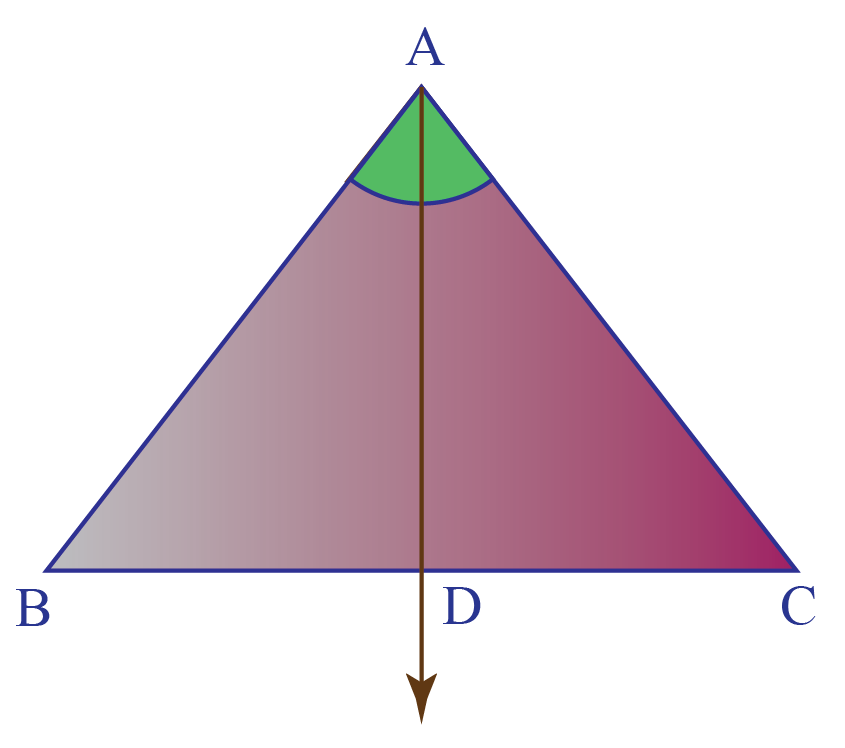Here, AD is the angle bisector.

$$\therefore \dfrac{AB}{AC}=\dfrac{BD}{CD}$$

Download Triangles and Quadrilaterals Worksheets
Triangles and Quadrilaterals
grade 9 | Questions Set 1
Triangles and Quadrilaterals
grade 9 | Answers Set 1
Triangles and Quadrilaterals
grade 9 | Questions Set 2
Triangles and Quadrilaterals
grade 9 | Answers Set 2

More Important Topics
Numbers
Algebra
Geometry
Measurement
Money
Data
Trigonometry
Calculus